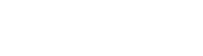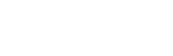# Bayes Rule

Bayes Rule is one of the most important theorems of Probability and lies in the heart of it. Keep reading to learn more about the Bayes Rule.

## Definition

Bayes rule (also known as Bayes theorem) gives the conditional probability of an event; that is, it describes the probability of an event, based on prior knowledge of conditions that might be related to the event. Bayes Rule is named after Reverend Thomas Bayes, who first provided an equation that allows new evidence to update beliefs in his ‘An Essay towards solving a Problem in the Doctrine of Chances’ (1763).

## Some Important Terms

Event – An event is simply the outcome of an experiment. For example, if you pick out a card and get a queen of spades, it is an event.

Sample Space – The sample space is the collection of all possible outcomes. Taking the previous example of cards, the sample space would be all 52 cards.

Union of Events – The union of two or more events is basically the combined set of the two or more events in the sample space. For example, the union of getting a king or hearts in a deck of cards would include 16 cards (13 hearts and 3 kings). Note that we do not count the king of hearts twice.

Intersection of Events – The intersection of two or more events is the set of events that are common in both the events. For example, in a deck of cards, the intersection of getting a queen and getting hearts would be the queen of hearts.

## Equation

Mathematically, the Bayes rule can be written as:Here, P(A) and P(B) are the probabilities of event A and event B independent of each other. This is known as marginal probability.

P(B/A) is the probability of occurrence of event B given that event A has already occurred. It is a conditional probability.

P(A/B) is the probability of occurrence of event A given that event B has already occurred. It is also a conditional probability.

Another way to represent Bayes’ theorem is:P (A ꓵ B) would represent the intersection of two events, A and B.

## Example

Let us understand this theorem with the help of a simple example. Suppose there is a bag filled with chocolates. There are two shapes of chocolate – round and flat. Suppose there are 7 round and 8 flat chocolates. We would like to find out the probability of getting a round chocolate given that the we have already got a round chocolate out.

This can be solved by using the Bayes’ theorem in the following way:
P(1) = Probability of getting a round chocolate at the first pick = 7/15
P(2) = Probability of getting a round chocolate at second pick = 6/14
P(1 and 2) = ½*(6/14)
P(2/1) = P(1 and 2)/P(1) = 0.214/0.4667=0.4585

So the probability of getting a round chocolate on the second picking given that the first picking yielded a round chocolate is 45.85%.

This very simple example helps us understand the Bayes rule for an uncomplicated case. This theorem can be extended to many cases which are more complicated. Also, this theorem can be used in different ways to obtain interesting and meaningful results.

## Bayesian Inference

Bayesian inference is a method of statistical inference in which Bayes’ theorem is used to update the probability of a hypothesis as more evidence or information becomes available. Bayesian inference is an important technique in statistics, and especially in mathematical statistics. Bayesian updating is particularly important in the dynamic analysis of a sequence of data.

Let us try to understand how this method works. Suppose it is late evening and you want to know if the light bulb in a room is switched on or switched off. There is some light in the room but it could be because of the sunlight (as it is late evening). So, you assume a probability that the light bulb is on, say p(i). This is the initial probability that the light bulb is on. Now, you try to gather evidence that will tell you if the light bulb is on or not. You check the switch. If the switch is on, the light bulb is on. This is the evidence that alters p(i) to a more accurate value. So we finally get p(i/switch) that is the probability of light being on given that the switch is on.

Bayesian inference is an extremely powerful set of tools for modeling any random variable, such as the value of a regression parameter, a demographic statistic, a business KPI, or the part of speech of a word. We provide our understanding of a problem and some data, and in return get a quantitative measure of how certain we are of a particular fact.

## Conclusion

Bayes’ Theorem is a basic but very useful concept in data analytics. It can be used in many problems to that deal with probability. For example, if crime is related to area, then, using Bayes’ theorem, a person’s area of residence can be used to more accurately estimate the probability of the person being a criminal, compared to the assessment of the probability of being a criminal made without knowledge of the person’s area. More complicated problems can be further solved using the Bayes’ theorem as a basis.

The methods related to using Bayes’ theorem come under Bayesian Statistics where various methods like Bayesian inference, Probability trees, Bayes’ updating and Frequentist approach.

##### Comments are closed.

Magoosh blog comment policy: To create the best experience for our readers, we will only approve comments that are relevant to the article, general enough to be helpful to other students, concise, and well-written! 😄 Due to the high volume of comments across all of our blogs, we cannot promise that all comments will receive responses from our instructors.

We highly encourage students to help each other out and respond to other students' comments if you can!

If you are a Premium Magoosh student and would like more personalized service from our instructors, you can use the Help tab on the Magoosh dashboard. Thanks!